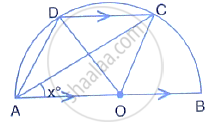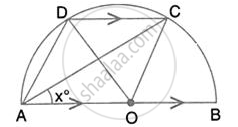Share

# In the Given Figure, Aob is a Diameter and Dc is Parallel to Ab. If ∠Cab = X°; Find (In Terms of X) the Values of ; ∠Cob, - Mathematics

Course

#### Question

In the given figure, AOB is a diameter and DC is parallel to AB. If ∠CAB = x°; find (in terms of x) the values of ;
∠COB,#### Solution∠COB = 2 ∠CAB = 2x

(Angle ate the centre is double the angler at the circumference subtended by the same order)

Is there an error in this question or solution?